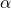# Cosine Function Introduction

In the circle above (which has radius 1), we see a radius from the pointto point A, and half of a chord, from the point A to the y axis. As we change the value of the angle, the length of the half chord changes. The study of the length of these half chords is the origin of the cosine function.

When, we see that the horizontal half chord has length 0.87.

We notice that in the first quadrant, this length is the same as the x coordinate of the point A.

We define the cosine of the angle as the x coordinate of the point A. Therefore,, (2 s.f.). Also,(2 s.f.).

The graph on the right maps the value ofto the x coordinate of the point A. Notice the point.

Move the slider to see how cosine changes (the x coordinate of point A) as the angle changes.

## Quick Comprehension Exercises:

You may use the applet above to help answer these questions.

1. What is the value of cos(60°)?
2. What is the value of cos(144°), correct to two decimal places?
3. Is the cosine of 272° positive or negative?4. In which quadrant is the angle 195°?
5. In which two quadrants is the value of cos(α°) negative?